Next: Sampled time and Nyquist Up: FOURIER SUMS Previous: FOURIER SUMS

## Superposition of sinusoids

Fourier analysis is built from the complex exponential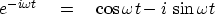(13)
A Fourier component of a time signal is a complex number, a sum of real and imaginary parts, say(14)
which is attached to some frequency. Let j be an integer and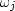be a set of frequencies. A signal b(t) can be manufactured by adding a collection of complex exponential signals, each complex exponential being scaled by a complex coefficient Bj, namely,(15)
This manufactures a complex-valued signal. How do we arrange for b(t) to be real? We can throw away the imaginary part, which is like adding b(t) to its complex conjugate,and then dividing by two:(16)
In other words, for each positivewith amplitude Bj, we add a negative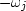with amplitude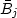(likewise, for every negative...). The Bj are called the frequency function,'' or the Fourier transform.'' Loosely, the Bj are called the spectrum,'' though technically, and in this book, the word spectrum'' should be reserved for the product.The words amplitude spectrum'' universally mean.

In practice, the collection of frequencies is almost always evenly spaced. Let j be an integer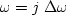so that(17)
Representing a signal by a sum of sinusoids is technically known as inverse Fourier transformation.'' An example of this is shown in Figure 6.cosines
Figure 6
Superposition of two sinusoids. (Press button to activate program ed1D. See appendix for details.)Next: Sampled time and Nyquist Up: FOURIER SUMS Previous: FOURIER SUMS
Stanford Exploration Project
10/21/1998